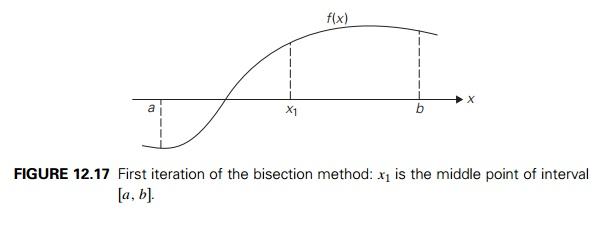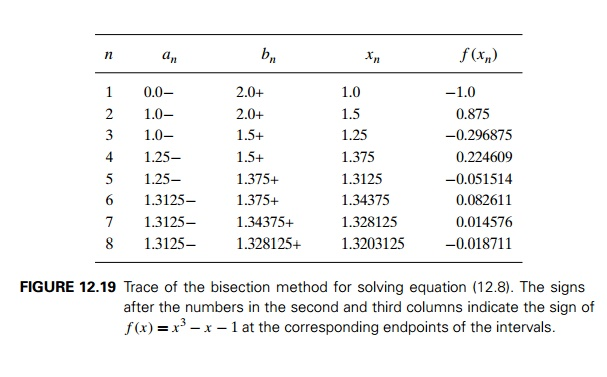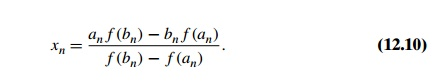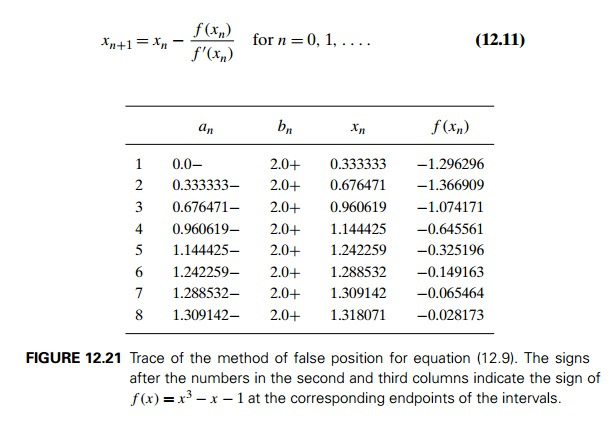Home | | Compiler Design | Algorithms for Solving Nonlinear Equations

# Algorithms for Solving Nonlinear Equations

In this section, we discuss several algorithms for solving nonlinear equations in one unknown,

Algorithms for Solving Nonlinear Equations

In this section, we discuss several algorithms for solving nonlinear equations in one unknown,There are several reasons for this choice among subareas of numerical analysis. First of all, this is an extremely important problem from both a practical and the-oretical point of view. It arises as a mathematical model of numerous phenomena in the sciences and engineering, both directly and indirectly. (Recall, for example, that the standard calculus technique for finding extremum points of a function f (x) is based on finding its critical points, which are the roots of the equation f (x) = 0.) Second, it represents the most accessible topic in numerical analysis and, at the same time, exhibits its typical tools and concerns. Third, some meth-ods for solving equations closely parallel algorithms for array searching and hence provide examples of applying general algorithm design techniques to problems of continuous mathematics.

Let us start with dispelling a misconception you might have about solving equations. Your experience with equation solving from middle school to calculus courses might have led you to believe that we can solve equations by “factoring” or by applying a readily available formula. Sorry to break it to you, but you have been deceived (with the best of educational intentions, of course): you were able to solve all those equations only because they had been carefully selected to make it possible. In general, we cannot solve equations exactly and need approximation algorithms to do so.

This is true even for solving the quadratic equation

ax2 + bx + c = 0

because the standard formula for its rootsrequires computing the square root, which can be done only approximately for most positive numbers. In addition, as we discussed in Section 11.4, this canonical formula needs to be modified to avoid the possibility of low-accuracy solutions.

What about formulas for roots of polynomials of degrees higher than two? Such formulas for third- and fourth-degree polynomials exist, but they are too cumbersome to be of practical value. For polynomials of degrees higher than four, there can be no general formula for their roots that would involve only the polynomial’s coefficients, arithmetical operations, and radicals (taking roots). This remarkable result was published first by the Italian mathematician and physician Paolo Ruffini (1765–1822) in 1799 and rediscovered a quarter century later by the Norwegian mathematician Niels Abel (1802–1829); it was developed further by the French mathematician Evariste Galois (1811–1832).4

The impossibility of such a formula can hardly be considered a great disap-pointment. As the great German mathematician Carl Friedrich Gauss (1777–1855) put it in his thesis of 1801, the algebraic solution of an equation was no better than devising a symbol for the root of the equation and then saying that the equation had a root equal to the symbol [OCo98].

We can interpret solutions to equation (12.4) as points at which the graph of the function f (x) intersects with the x-axis. The three algorithms we discuss in this section take advantage of this interpretation. Of course, the graph of f (x) may intersect the x-axis at a single point (e.g., x3 = 0), at multiple or even infinitely many points (sin x = 0), or at no point (e x + 1 = 0). Equation (12.4) would then have a single root, several roots, and no roots, respectively. It is a good idea to sketch a graph of the function before starting to approximate its roots. It can help to determine the number of roots and their approximate locations. In general, it is a good idea to isolate roots, i.e., to identify intervals containing a single root of the equation in question.

Bisection Method

This algorithm is based on an observation that the graph of a continuous function must intersect with the x-axis between two points a and b at least once if the function’s values have opposite signs at these two points (Figure 12.17).

The validity of this observation is proved as a theorem in calculus courses, and we take it for granted here. It serves as the basis of the following algorithm, called the bisection method, for solving equation (12.4). Starting with an interval [a, b] at whose endpoints f (x) has opposite signs, the algorithm computes the value of

f (x) at the middle point xmid = (a + b)/2. If f (xmid ) = 0, a root was found and the algorithm stops. Otherwise, it continues the search for a root either on [a, xmid ] or

on [xmid , b], depending on which of the two halves the values of f (x) have opposite signs at the endpoints of the new interval.

Since we cannot expect the bisection algorithm to stumble on the exact value of the equation’s root and stop, we need a different criterion for stopping the algo-rithm. We can stop the algorithm after the interval [an, bn] bracketing some root x becomes so small that we can guarantee that the absolute error of approximating x by xn, the middle point of this interval, is smaller than some small preselected number ε > 0. Since xn is the middle point of [an, bn] and x lies within this interval as well, we haveThis inequality implies that the sequence of approximations {xn} can be made as close to root x as we wish by choosing n large enough. In other words, we can say that {xn} converges to root x. Note, however, that because any digital computer represents extremely small values by zero (Section 11.4), the convergence asser-tion is true in theory but not necessarily in practice. In fact, if we choose ε below a certain machine-dependent threshold, the algorithm may never stop! Another source of potential complications is round-off errors in computing values of the function in question. Therefore, it is a good practice to include in a program im-plementing the bisection method a limit on the number of iterations the algorithm is allowed to run.

Here is pseudocode of the bisection method.

ALGORITHM     Bisection(f (x), a, b, eps, N )

//Implements the bisection method for finding a root of f (x) = 0 //Input: Two real numbers a and b, a < b,

a continuous function f (x) on [a, b], f (a)f (b) < 0,

an upper bound on the absolute error eps > 0,

an upper bound on the number of iterations N

//Output: An approximate (or exact) value x of a root in (a, b)

//or an interval bracketing the root if the iteration number limit is reached n 1 //iteration count

while n N do

x (a + b)/2

if x a < eps return x fval f (x)

if fval = 0 return x if fval f (a) < 0

b x else a x n n + 1

return “iteration limit”, a, b

Note that we can use inequality (12.7) to find in advance the number of iterations that should suffice, at least in theory, to achieve a preselected accuracy level. Indeed, choosing the number of iterations n large enough to satisfy (b1 a1)/2n < ε, i.e.,It has one real root. (See Figure 12.18 for the graph of f (x) = x3 x 1.) Since f (0) < 0 and f (2) > 0, the root must lie within interval [0, 2]. If we choose the error tolerance level as ε = 102, inequality (12.8) would require n > log2(2/102) or n 8 iterations.

Figure 12.19 contains a trace of the first eight iterations of the bisection method applied to equation (12.9).

Thus, we obtained x8 = 1.3203125 as an approximate value for the root x of equation (12.9), and we can guarantee that

|1.3203125x| < 102.

Moreover, if we take into account the signs of the function f (x) at a8, b8, and x8, we can assert that the root lies between 1.3203125 and 1.328125.

The principal weakness of the bisection method as a general algorithm for solving equations is its slow rate of convergence compared with other known methods. It is for this reason that the method is rarely used. Also, it cannot be extended to solving more general equations and systems of equations. But it does have several strong points. It always converges to a root whenever we start with aninterval whose properties are very easy to check. And it does not use derivatives of the function f (x) as some faster methods do.

What important algorithm does the method of bisection remind you of? If you have found it to closely resemble binary search, you are correct. Both of them solve variations of the searching problem, and they are both divide-by-half algorithms. The principal difference lies in the problem’s domain: discrete for binary search and continuous for the bisection method. Also note that while binary search requires its input array to be sorted, the bisection method does not require its function to be nondecreasing or nonincreasing. Finally, whereas binary search is very fast, the bisection method is relatively slow.Method of False Position

The method of false position (also known by its name in Latin, regula falsi) is to interpolation search as the bisection method is to binary search. Like the bisection method, it has, on each iteration, some interval [an, bn] bracketing a root of a continuous function f (x) that has opposite-sign values at an and bn. Unlike the bisection method, however, it computes the next root approximation not as the middle of [an, bn] but as the x-intercept of the straight line through the points (an, f (an)) and (bn, f (bn)) (Figure 12.20).

You are asked in the exercises to show that the formula for this x-intercept can be written asEXAMPLE 2 Figure 12.21 contains the results of the first eight iterations of this method for solving equation (12.9).

Although for this example the method of false position does not perform as well as the bisection method, for many instances it yields a faster converging sequence.

Newton’s method, also called the Newton-Raphson method, is one of the most im-portant general algorithms for solving equations. When applied to equation (12.4) in one unknown, it can be illustrated by Figure 12.22: the next element xn+1 of the method’s approximation sequence is obtained as the x-intercept of the tangent line to the graph of function f (x) at xn.

The analytical formula for the elements of the approximation sequence turns out to beIn most cases, Newton’s algorithm guarantees convergence of sequence (12.11) if an initial approximation x0 is chosen “close enough” to the root. (Precisely defined prescriptions for choosing x0 can be found in numerical analysis textbooks.) It may converge for initial approximations far from the root as well, but this is not always true.which is exactly the formula we used in Section 11.4 for computing approximate values of square roots.

EXAMPLE 4 Let us apply Newton’s method to equation (12.9), which we previ-ously solved with the bisection method and the method of false position. Formula (12.11) for this case becomesAs an initial element of the approximation sequence, we take, say, x0 = 2. Fig-ure 12.23 contains the results of the first five iterations of Newton’s method.

You cannot fail to notice how much faster Newton’s approximation sequence converges to the root than the approximation sequences of both the bisection method and the method of false position. This very fast convergence is typical of Newton’s method if an initial approximation is close to the equation’s root. Note, however, that on each iteration of this method we need to evaluate new values of the function and its derivative, whereas the previous two methods require only one new value of the function itself. Also, Newton’s method does not bracket a root as these two methods do. Moreover, for an arbitrary function and arbitrarily chosen initial approximation, its approximation sequence may diverge. And, because formula (12.11) has the function’s derivative in the denominator, the method may break down if it is equal to zero. In fact, Newton’s method is most effective when f (x) is bounded away from zero near root x. In particular, if

|f (x)| ≥ m1 > 0

on the interval between xn and x, we can estimate the distance between xn and x by using the Mean Value Theorem of calculus as follows:

f (xn) f (x) = f (c)(xn x),

where c is some point between xn and x. Since f (x) = 0 and |f (c)| ≥ m1, we obtainFormula (12.12) can be used as a criterion for stopping Newton’s algorithm when its right-hand side becomes smaller than a preselected accuracy level ε. Other possible stopping criteria arewhere ε is a small positive number. Since the last two criteria do not necessarily imply closeness of xn to root x, they should be considered inferior to the one based on (12.12).

The shortcomings of Newton’s method should not overshadow its principal strengths: fast convergence for an appropriately chosen initial approximation and applicability to much more general types of equations and systems of equations.

Exercises 12.4

a. Find on the Internet or in your library a procedure for finding a real root of the general cubic equation ax3 + bx2 + cx + d = 0 with real coefficients.

b. What general algorithm design technique is it based on?

Indicate how many roots each of the following equations has:

a. xex 1 = 0        b. x ln x = 0    c. x sin x 1 = 0

a. Prove that if p(x) is a polynomial of an odd degree, then it must have at least one real root.

Prove that if x0 is a root of an n-degree polynomial p(x), the polynomial can be factored into

p(x) = (x x0)q(x),

where q(x) is a polynomial of degree n 1. Explain what significance this theorem has for finding the roots of a polynomial.

c.  Prove that if x0 is a root of an n-degree polynomial p(x), then

p (x0) = q(x0),

where q(x) is the quotient of the division of p(x) by x x0.

Prove inequality (12.7).

Apply the bisection method to find the root of the equation

x3 + x 1 = 0

with an absolute error smaller than 102.

Derive formula (12.10) underlying the method of false position.

Apply the method of false position to find the root of the equation

x3 + x 1 = 0

with an absolute error smaller than 102.

Derive formula (12.11) underlying Newton’s method.

Apply Newton’s method to find the root of the equation

x3 + x 1 = 0

with an absolute error smaller than 102.

Give an example that shows that the approximation sequence of Newton’s method may diverge.

Gobbling goat There is a grassy field in the shape of a circle with a radius of 100 feet. A goat is attached by a rope to a hook at a fixed point on the field’s border. How long should the rope be to let the goat reach only half of the grass in the field?

SUMMARY

Backtracking and branch-and-bound are two algorithm design techniques for solving problems in which the number of choices grows at least exponentially with their instance size. Both techniques construct a solution one component at a time, trying to terminate the process as soon as one can ascertain that no solution can be obtained as a result of the choices already made. This approach makes it possible to solve many large instances of NP-hard problems in an acceptable amount of time.

Both backtracking and branch-and-bound employ, as their principal mech-anism, a state-space tree—a rooted tree whose nodes represent partially constructed solutions to the problem in question. Both techniques terminate a node as soon as it can be guaranteed that no solution to the problem can be obtained by considering choices that correspond to the node’s descendants.

Backtracking constructs its state-space tree in the depth-first-search fashion in the majority of its applications. If the sequence of choices represented by a current node of the state-space tree can be developed further without violating the problem’s constraints, it is done by considering the first remaining legitimate option for the next component. Otherwise, the method backtracks by undoing the last component of the partially built solution and replaces it by the next alternative.

Branch-and-bound is an algorithm design technique that enhances the idea of generating a state-space tree with the idea of estimating the best value obtainable from a current node of the decision tree: if such an estimate is not superior to the best solution seen up to that point in the processing, the node is eliminated from further consideration.

Approximation algorithms are often used to find approximate solutions to difficult problems of combinatorial optimization. The performance ratio is the principal metric for measuring the accuracy of such approximation algorithms.

The nearest-neighbor and multifragment heuristic are two simple greedy algorithms for approximating a solution to the traveling salesman problem. The performance ratios of these algorithms are unbounded above, even for the important subset of Euclidean graphs.

The twice-around-the-tree and Christofides algorithms exploit the graph’s minimum spanning tree to construct an Eulerian circuit and then transform it into a Hamiltonian circuit (an approximate solution to the TSP) by shortcuts. For Euclidean graphs, the performance ratios of these algorithms are 2 and 1.5, respectively.

Local search heuristics—the 2-opt, 3-opt, and Lin-Kernighan algorithms— work by replacing a few edges in the current tour to find a shorter one until no such replacement can be found. These algorithms are capable of finding in seconds a tour that is within a few percent of optimum for large Euclidean instances of the traveling salesman problem.

A sensible greedy algorithm for the knapsack problem is based on processing an input’s items in descending order of their value-to-weight ratios. For the continuous version of the problem, this algorithm always yields an exact optimal solution.

Polynomial approximation schemes for the knapsack problem are polynomial-time parametric algorithms that approximate solutions with any predefined accuracy level.

Solving nonlinear equations is one of the most important areas of numerical analysis. Although there are no formulas for roots of nonlinear equations (with a few exceptions), several algorithms can solve them approximately.

The bisection method and the method of false position are continuous analogues of binary search and interpolation search, respectively. Their principal advantage lies in bracketing a root on each iteration of the algorithm.

Newton’s method generates a sequence of root approximations that are x-intercepts of tangent lines to the function’s graph. With a good initial approximation, it typically requires just a few iterations to obtain a high-accuracy approximation to the equation’s root.

Study Material, Lecturing Notes, Assignment, Reference, Wiki description explanation, brief detail
Introduction to the Design and Analysis of Algorithms : Coping with the Limitations of Algorithm Power : Algorithms for Solving Nonlinear Equations |

Related Topics